Courses

# Test : Trigonometric Functions And Identities - 9

## 20 Questions MCQ Test Topic-wise Tests & Solved Examples for IIT JAM Mathematics | Test : Trigonometric Functions And Identities - 9

Description
This mock test of Test : Trigonometric Functions And Identities - 9 for Mathematics helps you for every Mathematics entrance exam. This contains 20 Multiple Choice Questions for Mathematics Test : Trigonometric Functions And Identities - 9 (mcq) to study with solutions a complete question bank. The solved questions answers in this Test : Trigonometric Functions And Identities - 9 quiz give you a good mix of easy questions and tough questions. Mathematics students definitely take this Test : Trigonometric Functions And Identities - 9 exercise for a better result in the exam. You can find other Test : Trigonometric Functions And Identities - 9 extra questions, long questions & short questions for Mathematics on EduRev as well by searching above.
QUESTION: 1

Solution:
QUESTION: 2

Solution:
QUESTION: 3

### The value of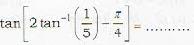Solution:
QUESTION: 4

Maximum value of sin x + cos x is.

Solution:
QUESTION: 5

The number of values of θ between 0 and 2π which satisfies the equation: sin4 θ - 2 sin2θ - 1 = 0

Solution:
QUESTION: 6

If log0.5 (x - 2) < log0.25(x- 2), then x lie s in the interval :

Solution:
QUESTION: 7

If log a, log b, log c are in A.P. then a, b, c are in

Solution:
QUESTION: 8

The greatest value of sin θ + cos θ is:

Solution:
QUESTION: 9

The equation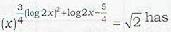Solution:
QUESTION: 10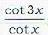never lies between :

Solution:
QUESTION: 11

The value of 4 cos 20° - √3 cot 20° is :

Solution:
QUESTION: 12

The number of roots of the equation x + 2 2tan x = π/2 lying between 0 and 2π is:

Solution:
QUESTION: 13

If tan β = 2 sinα sinγ cosec(a + y) then cotα, cotβ, cotγ are in:

Solution:
QUESTION: 14

If 2 cosθ = a + 1/a, then cos 3θ is equal to:

Solution:
QUESTION: 15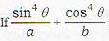is equal to:

Solution:
QUESTION: 16

The value of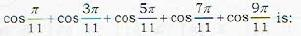Solution:
QUESTION: 17

The sum cos x + nc1 cos 2x + nc2 cos 3x + ... + nc3 cos(n + 1) x is equal to:

Solution:
QUESTION: 18

The value of cos248°-sin2 12° is:

Solution:
QUESTION: 19

Ifi n triangle ABC,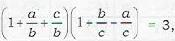then the angle A is equal to

Solution:
QUESTION: 20

For a triangle ABC,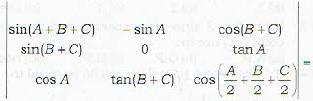Solution: